1592257440

# Model Complexity, Accuracy and Interpretability

Introduction
Complex Real-world challenges requires complex models to be build to give out predictions with utmost accuracy. However, they do not end up being highly interpretable. In this article, we will be looking into the relationship between complexity, accuracy and interpretability.
Motivation:
I am currently working as a Machine Learning Researcher and working on a project on Interpretable Machine Learning. This series is based of my implementation of the book by Christopher Molnar: “Interpretable Machine Learning”.

#model-interpretability #regression #data-science #machine-learning #python

## Buddha Community1596191340

## Uncovering the Magic: interpreting Machine Learning black-box models

**The trade-off between predictive power and interpretability **is a common issue to face when working with black-box models, especially in business environments where results have to be explained to non-technical audiences. Interpretability is crucial to being able to question, understand, and trust AI and ML systems. It also provides data scientists and engineers better means for debugging models and ensuring that they are working as intended.# Motivation

This tutorial aims to present different techniques for approaching model interpretation in black-box models.

_Disclaimer: _this article seeks to introduce some useful techniques from the field of interpretable machine learning to the average data scientists and to motivate its adoption . Most of them have been summarized from this highly recommendable book from Christoph Molnar: Interpretable Machine Learning.

The entire code used in this article can be found in my GitHub

# Contents

1. Taxonomy of Interpretability Methods
2. Dataset and Model Training
3. Global Importance
4. Local Importance

# 1. Taxonomy of Interpretability Methods

• **Intrinsic or Post-Hoc? **This criteria distinguishes whether interpretability is achieved by restricting the complexity of the machine learning model (intrinsic) or by applying methods that analyze the model after training (post-hoc).
• **Model-Specific or Model-Agnostic? **Linear models have a model-specific interpretation, since the interpretation of the regression weights are specific to that sort of models. Similarly, decision trees splits have their own specific interpretation. Model-agnostic tools, on the other hand, can be used on any machine learning model and are applied after the model has been trained (post-hoc).
• **Local or Global? **Local interpretability refers to explaining an individual prediction, whereas global interpretability is related to explaining the model general behavior in the prediction task. Both types of interpretations are important and there are different tools for addressing each of them.

# 2. Dataset and Model Training

Since the focus of this article is not centered in the modelling phase of the ML pipeline, minimum feature engineering was performed in order to model the data with an XGBoost.

The performance metrics obtained for the model are the following: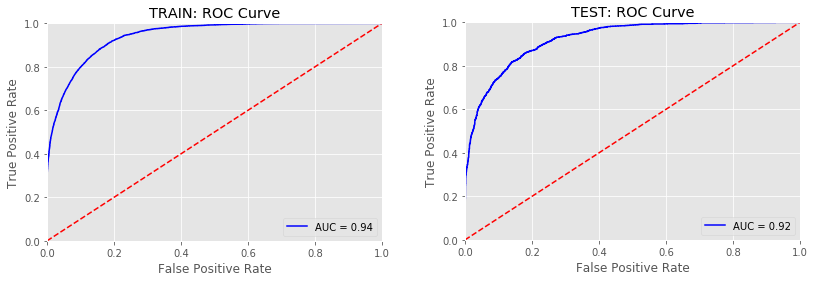Fig. 1: Receiving Operating Characteristic (ROC) curves for Train and Test sets.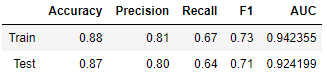Fig. 2: XGBoost performance metrics

The model’s performance seems to be pretty acceptable.

# 3. Global Importance

The techniques used to evaluate the global behavior of the model will be:

3.1 - Feature Importance (evaluated by the XGBoost model and by SHAP)

3.2 - Summary Plot (SHAP)

3.3 - Permutation Importance (ELI5)

3.4 - Partial Dependence Plot (PDPBox and SHAP)

3.5 - Global Surrogate Model (Decision Tree and Logistic Regression)

## 3.1 - Feature Importance

• XGBoost (model-specific)
``````feat_importances = pd.Series(clf_xgb_df.feature_importances_, index=X_train.columns).sort_values(ascending=True)
feat_importances.tail(20).plot(kind='barh')
``````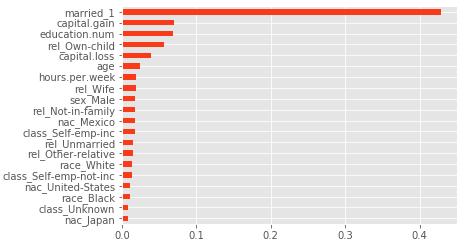Fig. 3: XGBoost Feature Importance

When working with XGBoost, one must be careful when interpreting features importances, since the results might be misleading. This is because the model calculates several importance metrics, with different interpretations. It creates an importance matrix, which is a table with the first column including the names of all the features actually used in the boosted trees, and the other with the resulting ‘importance’ values calculated with different metrics (Gain, Cover, Frequence). A more thourough explanation of these can be found here.

The **Gain **is the most relevant attribute to interpret the relative importance (i.e. improvement in accuracy) of each feature.

• SHAP

In general, SHAP library is considered to be a model-agnostic tool for addressing interpretability (we will cover SHAP’s intuition in the Local Importance section). However, the library has a model-specific method for tree-based machine learning models such as decision trees, random forests and gradient boosted trees.

``````explainer = shap.TreeExplainer(clf_xgb_df)
shap_values = explainer.shap_values(X_test)

shap.summary_plot(shap_values, X_test, plot_type = 'bar')
``````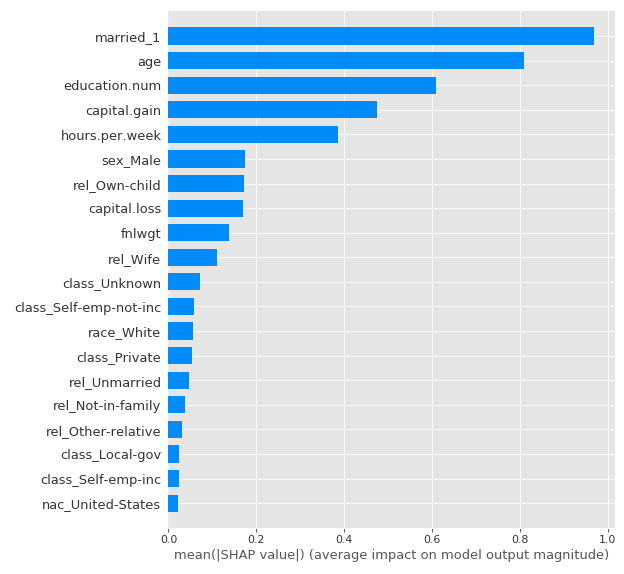Fig. 4: SHAP Feature Importance

The XGBoost feature importance was used to evaluate the relevance of the predictors in the model’s outputs for the Train dataset and the SHAP one to evaluate it for Test dataset, in order to assess if the most important features were similar in both approaches and sets.

It is observed that the most important variables of the model are maintained, although in different order of importance (age seems to take much more relevance in the test set by SHAP approach).

## 3.2 Summary Plot (SHAP)

The SHAP Summary Plot is a very interesting plot to evaluate the features of the model, since it provides more information than the traditional Feature Importance:

• Feature Importance: variables are sorted in descending order of importance.
• Impact on Prediction: the position on the horizontal axis indicates whether the values of the dataset instances for each feature have more or less impact on the output of the model.
• Original Value: the color indicates, for each feature, whether it is a high or low value (in the range of each of the feature).
• Correlation: the correlation of a feature with the model output can be analyzed by evaluating its color (its range of values) and the impact on the horizontal axis. For example, it is observed that the age has a positive correlation with the target, since the impact on the output increases as the value of the feature increases.
``````shap.summary_plot(shap_values, X_test)
``````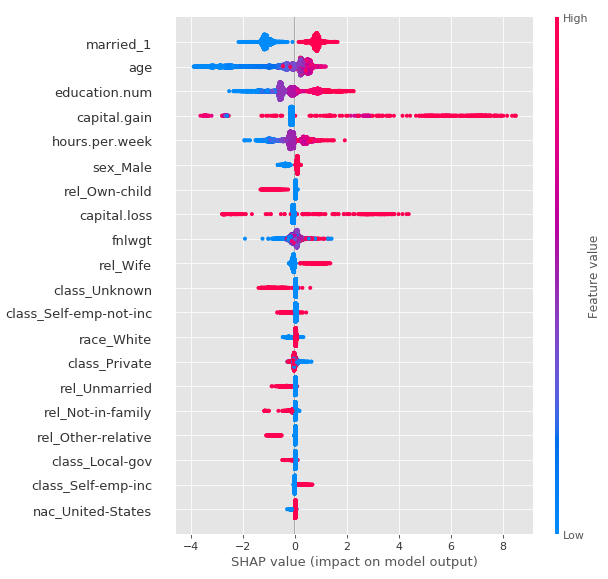Fig. 5: SHAP Summary Plot

## 3.3 - Permutation Importance (ELI5)

Another way to assess the global importance of the predictors is to randomly permute the order of the instances for each feature in the dataset and predict with the trained model. If by doing this disturbance in the order, the evaluation metric does not change substantially, then the feature is not so relevant. If instead the evaluation metric is affected, then the feature is considered important in the model. This process is done individually for each feature.

To evaluate the trained XGBoost model, the Area Under the Curve (AUC) of the ROC Curve will be used as the performance metric. Permutation Importance will be analyzed in both Train and Test:

``````# Train
perm = PermutationImportance(clf_xgb_df, scoring = 'roc_auc', random_state=1984).fit(X_train, y_train)
eli5.show_weights(perm, feature_names = X_train.columns.tolist())

# Test
perm = PermutationImportance(clf_xgb_df, scoring = 'roc_auc', random_state=1984).fit(X_test, y_test)
eli5.show_weights(perm, feature_names = X_test.columns.tolist())
``````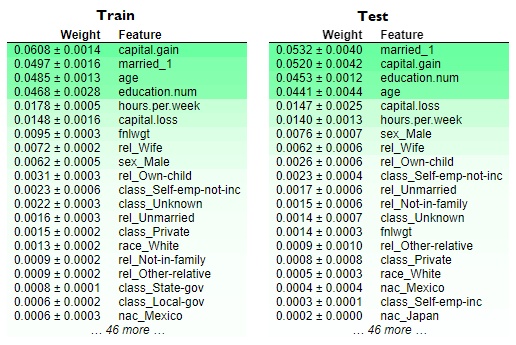Fig. 6: Permutation Importance for Train and Test sets.

Even though the order of the most important features changes, it looks like that the most relevant ones remain the same. It is interesting to note that, unlike the XGBoost Feature Importance, the age variable in the Train set has a fairly strong effect (as showed by SHAP Feature Importance in the Test set). Furthermore, the 6 most important variables according to the Permutation Importance are kept in Train and Test (the difference in order may be due to the distribution of each sample).

The coherence between the different approaches to approximate the global importance generates more confidence in the interpretation of the model’s output.

#model-interpretability #model-fairness #interpretability #machine-learning #shapley-values #deep learning1592257440

## Model Complexity, Accuracy and Interpretability

Introduction
Complex Real-world challenges requires complex models to be build to give out predictions with utmost accuracy. However, they do not end up being highly interpretable. In this article, we will be looking into the relationship between complexity, accuracy and interpretability.
Motivation:
I am currently working as a Machine Learning Researcher and working on a project on Interpretable Machine Learning. This series is based of my implementation of the book by Christopher Molnar: “Interpretable Machine Learning”.

#model-interpretability #regression #data-science #machine-learning #python1646789416

## Top 17 Machine Learning Algorithms with Scikit-Learn

### Machine Learning with Scikit-Learn

Scikit-learn is a library in Python that provides many unsupervised and supervised learning algorithms. It’s built upon some of the technology you might already be familiar with, like NumPy, pandas, and Matplotlib.

### The functionality that scikit-learn provides include:

• Regression, including Linear and Logistic Regression
• Classification, including K-Nearest Neighbors
• Clustering, including K-Means and K-Means++
• Model selection
• Preprocessing, including Min-Max Normalization

In this Article I will explain all machine learning algorithms with scikit-learn which you need to learn as a Data Scientist.

Lets start by importing the libraries:

``````%matplotlib inline
import numpy as np
import pandas as pd
import matplotlib.pyplot as plt
import seaborn as sns
from sklearn.model_selection import train_test_split
from sklearn.metrics import classification_report
from sklearn.linear_model import LinearRegression
from sklearn.metrics import accuracy_score
from sklearn.metrics import confusion_matrix
from scipy import stats
import pylab as pl``````

### Estimator

Given a scikit-learn estimator object named model, the following methods are available:

#### Available in all Estimators

model.fit() : fit training data. For supervised learning applications, this accepts two arguments: the data X and the labels y (e.g. model.fit(X, y)). For unsupervised learning applications, this accepts only a single argument, the data X (e.g. model.fit(X)).

#### Available in supervised estimators

model.predict() : given a trained model, predict the label of a new set of data. This method accepts one argument, the new data X_new (e.g. model.predict(X_new)), and returns the learned label for each object in the array.

model.predict_proba() : For classification problems, some estimators also provide this method, which returns the probability that a new observation has each categorical label. In this case, the label with the highest probability is returned by model.predict(). model.score() : for classification or regression problems, most (all?) estimators implement a score method. Scores are between 0 and 1, with a larger score indicating a better fit.

#### Available in unsupervised estimators

model.predict() : predict labels in clustering algorithms. model.transform() : given an unsupervised model, transform new data into the new basis. This also accepts one argument X_new, and returns the new representation of the data based on the unsupervised model. model.fit_transform() : some estimators implement this method, which more efficiently performs a fit and a transform on the same input data.

``````data =  pd.read_csv('Iris.csv')
``print(data.shape)``
``````#Output
(150, 6)``````
``data.info()``
``````#Output
<class 'pandas.core.frame.DataFrame'>
RangeIndex: 150 entries, 0 to 149
Data columns (total 6 columns):
#   Column         Non-Null Count  Dtype
---  ------         --------------  -----
0   Id             150 non-null    int64
1   SepalLengthCm  150 non-null    float64
2   SepalWidthCm   150 non-null    float64
3   PetalLengthCm  150 non-null    float64
4   PetalWidthCm   150 non-null    float64
5   Species        150 non-null    object
dtypes: float64(4), int64(1), object(1)
memory usage: 7.2+ KB``````

### Visualization

Some graphical representation of information and data.

``````sns.FacetGrid(data,hue='Species',size=5)\
.map(plt.scatter,'SepalLengthCm','SepalWidthCm')\
``sns.pairplot(data,hue='Species')``

### Prepare Train and Test

scikit-learn provides a helpful function for partitioning data, train_test_split, which splits out your data into a training set and a test set.

Training and test usually is 70% for training and 30% for test

• Training set for fitting the model
• Test set for evaluation only
``````X = data.iloc[:, :-1].values    #   X -> Feature Variables
y = data.iloc[:, -1].values #   y ->  Target
# Splitting the data into Train and Test
X_train, X_test, y_train, y_test = train_test_split(X, y, test_size = 0.3, random_state = 0)``````

### Algorithm 1 – Linear Regression

It is used to estimate real values (cost of houses, number of calls, total sales etc.) based on continuous variable(s). Here, we establish relationship between independent and dependent variables by fitting a best line. This best fit line is known as regression line and represented by a linear equation **Y= a *X + b.

``````#converting object data type into int data type using labelEncoder for Linear reagration in this case

XL = data.iloc[:, :-1].values    #   X -> Feature Variables
yL = data.iloc[:, -1].values #   y ->  Target

from sklearn.preprocessing import LabelEncoder

le = LabelEncoder()
Y_train= le.fit_transform(yL)

print(Y_train)  # this is Y_train categotical to numerical
``````
``````#Output
[0 0 0 0 0 0 0 0 0 0 0 0 0 0 0 0 0 0 0 0 0 0 0 0 0 0 0 0 0 0 0 0 0 0 0 0 0
0 0 0 0 0 0 0 0 0 0 0 0 0 1 1 1 1 1 1 1 1 1 1 1 1 1 1 1 1 1 1 1 1 1 1 1 1
1 1 1 1 1 1 1 1 1 1 1 1 1 1 1 1 1 1 1 1 1 1 1 1 1 1 2 2 2 2 2 2 2 2 2 2 2
2 2 2 2 2 2 2 2 2 2 2 2 2 2 2 2 2 2 2 2 2 2 2 2 2 2 2 2 2 2 2 2 2 2 2 2 2
2 2]``````
``````# This is only for Linear Regretion
X_trainL, X_testL, y_trainL, y_testL = train_test_split(XL, Y_train, test_size = 0.3, random_state = 0)``````
``````from sklearn.linear_model import LinearRegression
modelLR = LinearRegression()
modelLR.fit(X_trainL, y_trainL)
Y_pred = modelLR.predict(X_testL)``````
``````from sklearn import metrics
#calculating the residuals
print('y-intercept             :' , modelLR.intercept_)
print('beta coefficients       :' , modelLR.coef_)
print('Mean Abs Error MAE      :' ,metrics.mean_absolute_error(y_testL,Y_pred))
print('Mean Sqrt Error MSE     :' ,metrics.mean_squared_error(y_testL,Y_pred))
print('Root Mean Sqrt Error RMSE:' ,np.sqrt(metrics.mean_squared_error(y_testL,Y_pred)))
print('r2 value                :' ,metrics.r2_score(y_testL,Y_pred))``````
``````#Output
y-intercept             : -0.024298523519848292
beta coefficients       : [ 0.00680677 -0.10726764 -0.00624275  0.22428158  0.27196685]
Mean Abs Error MAE      : 0.14966835490524963
Mean Sqrt Error MSE     : 0.03255451737969812
Root Mean Sqrt Error RMSE: 0.18042870442282213
r2 value                : 0.9446026069799255``````

### Algorithm 2- Decision Tree

This is one of my favorite algorithm and I use it quite frequently. It is a type of supervised learning algorithm that is mostly used for classification problems. Surprisingly, it works for both categorical and continuous dependent variables.

In this algorithm, we split the population into two or more homogeneous sets. This is done based on most significant attributes/ independent variables to make as distinct groups as possible.

``````# Decision Tree's
from sklearn.tree import DecisionTreeClassifier

Model = DecisionTreeClassifier()

Model.fit(X_train, y_train)

y_pred = Model.predict(X_test)

# Summary of the predictions made by the classifier
print(classification_report(y_test, y_pred))
print(confusion_matrix(y_test, y_pred))
# Accuracy score
print('accuracy is',accuracy_score(y_pred,y_test))``````
``````#Output
precision    recall  f1-score   support

Iris-setosa       1.00      1.00      1.00        16
Iris-versicolor       0.95      1.00      0.97        18
Iris-virginica       1.00      0.91      0.95        11

accuracy                           0.98        45
macro avg       0.98      0.97      0.98        45
weighted avg       0.98      0.98      0.98        45

[[16  0  0]
[ 0 18  0]
[ 0  1 10]]
accuracy is 0.9777777777777777``````

## Algorithm 3- RandomForest

Random Forest is a trademark term for an ensemble of decision trees. In Random Forest, we’ve collection of decision trees (so known as “Forest”). To classify a new object based on attributes, each tree gives a classification and we say the tree “votes” for that class. The forest chooses the classification having the most votes (over all the trees in the forest).

``````from sklearn.ensemble import RandomForestClassifier
Model=RandomForestClassifier(max_depth=2)
Model.fit(X_train,y_train)
y_pred=Model.predict(X_test)

# Summary of the predictions made by the classifier
print(classification_report(y_test,y_pred))
print(confusion_matrix(y_pred,y_test))
#Accuracy Score
print('accuracy is ',accuracy_score(y_pred,y_test))``````
``````#Output
precision    recall  f1-score   support

Iris-setosa       1.00      1.00      1.00        16
Iris-versicolor       1.00      1.00      1.00        18
Iris-virginica       1.00      1.00      1.00        11

accuracy                           1.00        45
macro avg       1.00      1.00      1.00        45
weighted avg       1.00      1.00      1.00        45

[[16  0  0]
[ 0 18  0]
[ 0  0 11]]
accuracy is  1.0``````

### Algorithm 4- Logistic Regression

Don’t get confused by its name! It is a classification not a regression algorithm. It is used to estimate discrete values ( Binary values like 0/1, yes/no, true/false ) based on given set of independent variable(s).

In simple words, it predicts the probability of occurrence of an event by fitting data to a logic function. Hence, it is also known as logic regression. Since, it predicts the probability, its output values lies between 0 and 1 (as expected).

``````# LogisticRegression
from sklearn.linear_model import LogisticRegression
Model = LogisticRegression()
Model.fit(X_train, y_train)

y_pred = Model.predict(X_test)

# Summary of the predictions made by the classifier
print(classification_report(y_test, y_pred))
print(confusion_matrix(y_test, y_pred))
# Accuracy score
print('accuracy is',accuracy_score(y_pred,y_test))``````
``````#Output
precision    recall  f1-score   support

Iris-setosa       1.00      1.00      1.00        16
Iris-versicolor       1.00      1.00      1.00        18
Iris-virginica       1.00      1.00      1.00        11

accuracy                           1.00        45
macro avg       1.00      1.00      1.00        45
weighted avg       1.00      1.00      1.00        45

[[16  0  0]
[ 0 18  0]
[ 0  0 11]]
accuracy is 1.0``````

### Algorithm 5- K Nearest Neighbors

It can be used for both classification and regression problems. However, it is more widely used in classification problems in the industry. K nearest neighbors is a simple algorithm that stores all available cases and classifies new cases by a majority vote of its k neighbors. The case being assigned to the class is most common amongst its K nearest neighbors measured by a distance function.

``````# K-Nearest Neighbours
from sklearn.neighbors import KNeighborsClassifier

Model = KNeighborsClassifier(n_neighbors=8)
Model.fit(X_train, y_train)

y_pred = Model.predict(X_test)

# Summary of the predictions made by the classifier
print(classification_report(y_test, y_pred))
print(confusion_matrix(y_test, y_pred))
# Accuracy score

print('accuracy is',accuracy_score(y_pred,y_test))``````
``````#Output
precision    recall  f1-score   support

Iris-setosa       1.00      1.00      1.00        16
Iris-versicolor       1.00      1.00      1.00        18
Iris-virginica       1.00      1.00      1.00        11

accuracy                           1.00        45
macro avg       1.00      1.00      1.00        45
weighted avg       1.00      1.00      1.00        45

[[16  0  0]
[ 0 18  0]
[ 0  0 11]]
accuracy is 1.0``````

### Algorithm 6- Naive Bayes

It is a classification technique based on Bayes’ theorem with an assumption of independence between predictors. In simple terms, a Naive Bayes classifier assumes that the presence of a particular feature in a class is unrelated to the presence of any other feature.

For example, a fruit may be considered to be an apple if it is red, round, and about 3 inches in diameter. Even if these features depend on each other or upon the existence of the other features, a naive Bayes classifier would consider all of these properties to independently contribute to the probability that this fruit is an apple.

``````# Naive Bayes
from sklearn.naive_bayes import GaussianNB
Model = GaussianNB()
Model.fit(X_train, y_train)

y_pred = Model.predict(X_test)

# Summary of the predictions made by the classifier
print(classification_report(y_test, y_pred))
print(confusion_matrix(y_test, y_pred))
# Accuracy score
print('accuracy is',accuracy_score(y_pred,y_test))``````
``````#Output
precision    recall  f1-score   support

Iris-setosa       1.00      1.00      1.00        16
Iris-versicolor       1.00      1.00      1.00        18
Iris-virginica       1.00      1.00      1.00        11

accuracy                           1.00        45
macro avg       1.00      1.00      1.00        45
weighted avg       1.00      1.00      1.00        45

[[16  0  0]
[ 0 18  0]
[ 0  0 11]]
accuracy is 1.0``````

### Algorithm 7- Support Vector Machines

It is a classification method. In this algorithm, we plot each data item as a point in n-dimensional space (where n is number of features you have) with the value of each feature being the value of a particular coordinate.

For example, if we only had two features like Height and Hair length of an individual, we’d first plot these two variables in two dimensional space where each point has two co-ordinates (these co-ordinates are known as Support Vectors)

``````# Support Vector Machine
from sklearn.svm import SVC

Model = SVC()
Model.fit(X_train, y_train)

y_pred = Model.predict(X_test)

# Summary of the predictions made by the classifier
print(classification_report(y_test, y_pred))
print(confusion_matrix(y_test, y_pred))
# Accuracy score

print('accuracy is',accuracy_score(y_pred,y_test))``````
``````#Output
precision    recall  f1-score   support

Iris-setosa       1.00      1.00      1.00        16
Iris-versicolor       1.00      1.00      1.00        18
Iris-virginica       1.00      1.00      1.00        11

accuracy                           1.00        45
macro avg       1.00      1.00      1.00        45
weighted avg       1.00      1.00      1.00        45

[[16  0  0]
[ 0 18  0]
[ 0  0 11]]
accuracy is 1.0``````

### Algorithm 8- Radius Neighbors Classifier

In scikit-learn RadiusNeighborsClassifier is very similar to KNeighborsClassifier with the exception of two parameters. First, in RadiusNeighborsClassifier we need to specify the radius of the fixed area used to determine if an observation is a neighbor using radius.

Unless there is some substantive reason for setting radius to some value, it is best to treat it like any other hyperparameter and tune it during model selection. The second useful parameter is outlier_label, which indicates what label to give an observation that has no observations within the radius – which itself can often be a useful tool for identifying outliers.

``````#Output
Model.fit(X_train,y_train)
y_pred=Model.predict(X_test)

#summary of the predictions made by the classifier
print(classification_report(y_test,y_pred))
print(confusion_matrix(y_test,y_pred))

#Accouracy score
print('accuracy is ', accuracy_score(y_test,y_pred))``````
``````#Output
precision    recall  f1-score   support

Iris-setosa       1.00      1.00      1.00        16
Iris-versicolor       1.00      1.00      1.00        18
Iris-virginica       1.00      1.00      1.00        11

accuracy                           1.00        45
macro avg       1.00      1.00      1.00        45
weighted avg       1.00      1.00      1.00        45

[[16  0  0]
[ 0 18  0]
[ 0  0 11]]
accuracy is  1.0``````

### Algorithm 9- Passive Aggressive Classifier

PA algorithm is a margin based online learning algorithm for binary classification. Unlike PA algorithm, which is a hard-margin based method, PA-I algorithm is a soft margin based method and robuster to noise.

``````from sklearn.linear_model import PassiveAggressiveClassifier
Model = PassiveAggressiveClassifier()
Model.fit(X_train, y_train)

y_pred = Model.predict(X_test)

# Summary of the predictions made by the classifier
print(classification_report(y_test, y_pred))
print(confusion_matrix(y_test, y_pred))
# Accuracy score
print('accuracy is',accuracy_score(y_pred,y_test))``````
``````#Output
precision    recall  f1-score   support

Iris-setosa       0.89      1.00      0.94        16
Iris-versicolor       0.00      0.00      0.00        18
Iris-virginica       0.41      1.00      0.58        11

accuracy                           0.60        45
macro avg       0.43      0.67      0.51        45
weighted avg       0.42      0.60      0.48        45

[[16  0  0]
[ 2  0 16]
[ 0  0 11]]
accuracy is 0.6``````

## Algorithm 10- BernoulliNB

Like MultinomialNB, this classifier is suitable for discrete data. The difference is that while MultinomialNB works with occurrence counts, BernoulliNB is designed for binary/boolean features.

``````# BernoulliNB
from sklearn.naive_bayes import BernoulliNB
Model = BernoulliNB()
Model.fit(X_train, y_train)

y_pred = Model.predict(X_test)

# Summary of the predictions made by the classifier
print(classification_report(y_test, y_pred))
print(confusion_matrix(y_test, y_pred))
# Accuracy score
print('accuracy is',accuracy_score(y_pred,y_test))``````
``````#Output
precision    recall  f1-score   support

Iris-setosa       0.00      0.00      0.00        16
Iris-versicolor       0.00      0.00      0.00        18
Iris-virginica       0.24      1.00      0.39        11

accuracy                           0.24        45
macro avg       0.08      0.33      0.13        45
weighted avg       0.06      0.24      0.10        45

[[ 0  0 16]
[ 0  0 18]
[ 0  0 11]]
accuracy is 0.24444444444444444``````

### Algorithm 11- ExtraTreeClassifier

ExtraTreesClassifier is an ensemble learning method fundamentally based on decision trees. ExtraTreesClassifier, like RandomForest, randomizes certain decisions and subsets of data to minimize over-learning from the data and overfitting. Let’s look at some ensemble methods ordered from high to low variance, ending in ExtraTreesClassifier.

``````# ExtraTreeClassifier
from sklearn.tree import ExtraTreeClassifier

Model = ExtraTreeClassifier()

Model.fit(X_train, y_train)

y_pred = Model.predict(X_test)

# Summary of the predictions made by the classifier
print(classification_report(y_test, y_pred))
print(confusion_matrix(y_test, y_pred))
# Accuracy score
print('accuracy is',accuracy_score(y_pred,y_test))``````
``````#Output
precision    recall  f1-score   support

Iris-setosa       1.00      1.00      1.00        16
Iris-versicolor       1.00      0.94      0.97        18
Iris-virginica       0.92      1.00      0.96        11

accuracy                           0.98        45
macro avg       0.97      0.98      0.98        45
weighted avg       0.98      0.98      0.98        45

[[16  0  0]
[ 0 17  1]
[ 0  0 11]]
accuracy is 0.9777777777777777``````

### Algorithm 12- Bagging classifier

Bagging classifier is an ensemble meta-estimator that fits base classifiers each on random subsets of the original dataset and then aggregate their individual predictions (either by voting or by averaging) to form a final prediction. Such a meta-estimator can typically be used as a way to reduce the variance of a black-box estimator (e.g., a decision tree), by introducing randomization into its construction procedure and then making an ensemble out of it.

``````#Output
precision    recall  f1-score   support

Iris-setosa       1.00      1.00      1.00        16
Iris-versicolor       0.95      1.00      0.97        18
Iris-virginica       1.00      0.91      0.95        11

accuracy                           0.98        45
macro avg       0.98      0.97      0.98        45
weighted avg       0.98      0.98      0.98        45

[[16  0  0]
[ 0 18  1]
[ 0  0 10]]
accuracy is  0.9777777777777777``````

An AdaBoost classifier is a meta-estimator that begins by fitting a classifier on the original dataset and then fits additional copies of the classifier on the same dataset but where the weights of incorrectly classified instances are adjusted such that subsequent classifiers focus more on difficult cases.

``````from sklearn.ensemble import AdaBoostClassifier
Model.fit(X_train,y_train)
y_pred=Model.predict(X_test)

# Summary of the predictions made by the classifier
print(classification_report(y_test,y_pred))
print(confusion_matrix(y_pred,y_test))
#Accuracy Score
print('accuracy is ',accuracy_score(y_pred,y_test))``````
``````#Output
precision    recall  f1-score   support

Iris-setosa       1.00      1.00      1.00        16
Iris-versicolor       0.95      1.00      0.97        18
Iris-virginica       1.00      0.91      0.95        11

accuracy                           0.98        45
macro avg       0.98      0.97      0.98        45
weighted avg       0.98      0.98      0.98        45

[[16  0  0]
[ 0 18  1]
[ 0  0 10]]
accuracy is  0.9777777777777777``````

### Algorithm 14- Gradient Boosting Classifier

GBM is a boosting algorithm used when we deal with plenty of data to make a prediction with high prediction power. Boosting is actually an ensemble of learning algorithms which combines the prediction of several base estimators in order to improve robustness over a single estimator. It combines multiple weak or average predictors to a build strong predictor.

``````from sklearn.ensemble import GradientBoostingClassifier
Model.fit(X_train,y_train)
y_pred=Model.predict(X_test)

# Summary of the predictions made by the classifier
print(classification_report(y_test,y_pred))
print(confusion_matrix(y_pred,y_test))

#Accuracy Score
print('accuracy is ',accuracy_score(y_pred,y_test))``````
``````#Output
precision    recall  f1-score   support

Iris-setosa       1.00      1.00      1.00        16
Iris-versicolor       0.95      1.00      0.97        18
Iris-virginica       1.00      0.91      0.95        11

accuracy                           0.98        45
macro avg       0.98      0.97      0.98        45
weighted avg       0.98      0.98      0.98        45

[[16  0  0]
[ 0 18  1]
[ 0  0 10]]
accuracy is  0.9777777777777777``````

### Algorithm 15- Linear Discriminant Analysis

A classifier with a linear decision boundary, generated by fitting class conditional densities to the data and using Bayes’ rule.

The model fits a Gaussian density to each class, assuming that all classes share the same covariance matrix.

The fitted model can also be used to reduce the dimensionality of the input by projecting it to the most discriminative directions.

``````from sklearn.discriminant_analysis import LinearDiscriminantAnalysis
Model=LinearDiscriminantAnalysis()
Model.fit(X_train,y_train)
y_pred=Model.predict(X_test)

# Summary of the predictions made by the classifier
print(classification_report(y_test,y_pred))
print(confusion_matrix(y_pred,y_test))

#Accuracy Score
print('accuracy is ',accuracy_score(y_pred,y_test))``````
``````#Output
precision    recall  f1-score   support

Iris-setosa       1.00      1.00      1.00        16
Iris-versicolor       1.00      1.00      1.00        18
Iris-virginica       1.00      1.00      1.00        11

accuracy                           1.00        45
macro avg       1.00      1.00      1.00        45
weighted avg       1.00      1.00      1.00        45

[[16  0  0]
[ 0 18  0]
[ 0  0 11]]
accuracy is  1.0``````

### Algorithm 16- Quadratic Discriminant Analysis

A classifier with a quadratic decision boundary, generated by fitting class conditional densities to the data and using Bayes’ rule.

The model fits a Gaussian density to each class.

``````#Output
precision    recall  f1-score   support

Iris-setosa       1.00      1.00      1.00        16
Iris-versicolor       1.00      1.00      1.00        18
Iris-virginica       1.00      1.00      1.00        11

accuracy                           1.00        45
macro avg       1.00      1.00      1.00        45
weighted avg       1.00      1.00      1.00        45

[[16  0  0]
[ 0 18  0]
[ 0  0 11]]
accuracy is  1.0``````

### Algorithm 17- K- means

It is a type of unsupervised algorithm which solves the clustering problem. Its procedure follows a simple and easy way to classify a given data set through a certain number of clusters (assume k clusters). Data points inside a cluster are homogeneous and heterogeneous to peer groups.

Remember figuring out shapes from ink blots? k means is somewhat similar this activity. You look at the shape and spread to decipher how many different clusters / population are present.

``````x = data.iloc[:, [1, 2, 3, 4]].values

#Finding the optimum number of clusters for k-means classification
from sklearn.cluster import KMeans
wcss = []

for i in range(1, 11):
kmeans = KMeans(n_clusters = i, init = 'k-means++', max_iter = 300, n_init = 10, random_state = 0)
kmeans.fit(x)
wcss.append(kmeans.inertia_)

#Plotting the results onto a line graph, allowing us to observe 'The elbow'
plt.plot(range(1, 11), wcss)
plt.title('The elbow method')
plt.xlabel('Number of clusters')
plt.ylabel('WCSS') # within cluster sum of squares
plt.show()``````
``````#Applying kmeans to the dataset / Creating the kmeans classifier
kmeans = KMeans(n_clusters = 3, init = 'k-means++', max_iter = 300, n_init = 10, random_state = 0)
y_kmeans = kmeans.fit_predict(x)
#Visualising the clusters

plt.scatter(x[y_kmeans == 0, 0], x[y_kmeans == 0, 1], s = 100, c = 'red', label = 'Iris-Setosa')
plt.scatter(x[y_kmeans == 1, 0], x[y_kmeans == 1, 1], s = 100, c = 'blue', label = 'Iris-Versicolour')
plt.scatter(x[y_kmeans == 2, 0], x[y_kmeans == 2, 1], s = 100, c = 'yellow', label = 'Iris-Virginica')

#Plotting the centroids of the clusters
plt.scatter(kmeans.cluster_centers_[:, 0], kmeans.cluster_centers_[:,1], s = 100, c = 'green', label = 'Centroids',marker='*')

plt.legend()``````

Original article source at https://thecleverprogrammer.com

#machinelearning #algorithms1592229900

## Model Complexity, Accuracy and Interpretability

Introduction
Complex Real-world challenges requires complex models to be build to give out predictions with utmost accuracy. However, they do not end up being highly interpretable. In this article, we will be looking into the relationship between complexity, accuracy and interpretability.
Motivation:
I am currently working as a Machine Learning Researcher and working on a project on Interpretable Machine Learning. This series is based of my implementation of the book by Christopher Molnar: “Interpretable Machine Learning”.

#model-interpretability #regression #data-science #machine-learning #python1593972060

## Interpreting the model is for humans, not for computers

Critique of pure interpretation
The scientific method as the tool that has served us to find explanations about how things work and make decisions, brought us the biggest challenge that until 2020 we have probably still not overcome: Giving useful narratives to numbers. Also known as “interpretation”.
Just as a matter of clarification, the scientific method is the pipeline of finding evidence to prove or disprove hypotheses. Science and how things work is everything, from natural sciences to economic sciences. But most delightful, by evidence, not only we mean, but humanity understands “data”. And data cannot be anything less than numbers.
Leaving space for generality, the problem of interpretation is particularly entertaining along the path of statistical analyses within the scientific method pipeline. This means finding models that are written in a mathematical language and finding an interpretation for them within the context that delivered the data.
Interpreting a model has two crucial implications that many scientists or science technicians have for long skipped (hopefully not forgotten). The first one relies on the fact that if there is a model to interpret now, there must have been a research question asked before in a context that delivered data to build such a model. The second one is that the narratives we need to create about our model can do much more by expressing ideas about a number within the context of the research question rather than purely inside the model. After all and until 2020, the decisions are made by humans based on the meaning of those numbers, not really by computers. And this last statement is important, because in the 21st century we might actually get to the point that computers take over us in many tasks and they might end up making decisions for us. For this they will need to communicate those decisions among their network. Just then, the human narratives will not count since computers only understand numbers.
As statisticians, we have been adopting the practice of finding problems to solve, finding questions to answer and answers to explain using available data. This mindset has kept us running on a circle of non-sense narratives and interpretations because problems are not found or looked for. Problems and questions emerge from all ongoing interactions and reactions of different phenomena. This fact implies that statistical models and/or other analytical approaches are tools to be used upon the core central problem or question, they are not the spine.
This ugly art of fitting a linear regression on some data and saying that “the beta coefficient is the amount of units that y increases when x increases one unit”, or the art of calculating an average and saying that “it is the value around which we can find the majority of the data points” is a ruthless product that we statisticians have been offering to the scientific method.
The bubble of interpretation
Teaching statistics has made clear for us that people can perfectly understand the way the models work and how to train them to get the numbers. However, what we still did not digest is the fact that out of all the numbers that are produced when training models, most are simply noncommunicable for non statistical people. Let us present some of these numbers whose communication is dark:

• The p-value is one particular concept that may in fact deserve an entire essay on its own. On social media, for instance, we constantly see people asking about an explanation of the p-value and right away there is a storm of statisticians engaging into giving their own interpretation.
• The odds-ratio stars in this debate. After 10 years of working in this field, we must admit that it has never been possible to explain to an expert in another field how to think about the odds ratio. Even Wikipedia has tried out and, in our opinion, has more than failed at it.
• The beta coefficients of a dummy variable in a logistic regression are of the same kind. We have the feeling that they are odds-ratio of the categories with respect to the baseline category. But, how can we make it understandable and actionable in practice? This simply we don’t know.
This problem is central for the scientific and statistical community. The models that we train lose their value because of such a lack of interpretation.
After some years of talking with colleagues about this problem and looking for an appropriate framework that clears up the problem of interpretation, we had come to one obvious conclusion: the interpretation process exists and can only happen within a given context. It makes no sense to fight for the interpretation process inside the model. The inner processes of the model are all numerical and these results can be communicated and understood only by numerical, statistical people. Making interpretations of numbers inside the models is a bubble of rephrasing. In order to interpret the results of a model so that they become tools for taking actions, it is essential to keep in mind the context from where the data is coming and the research questions are asked.

#interpretation #model-interpretability #semantics #data #data-analysis #data analysis